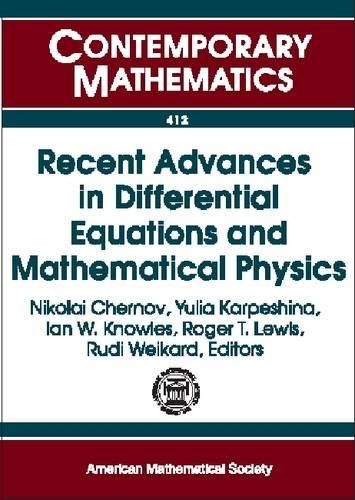•# Recent Advances in Differential Equations and Mathematical Physics - Contemporary Mathematics (Paperback)

(editor), (editor), (editor), (editor), (editor)
£101.00
Paperback 333 Pages / Published: 30/08/2006
• We can order this

Usually dispatched within 3 weeks

This book brings together both new material and recent surveys on some topics in differential equations that are either directly relevant to, or closely associated with, mathematical physics. Its topics include asymptotic formulas for the ground-state energy of fermionic gas, renormalization ideas in quantum field theory from perturbations of the free Hamiltonian on the circle, \$J\$-self adjoint Dirac operators, spectral theory of Schrodinger operators, inverse problems, isoperimetric inequalities in quantum mechanics, Hardy inequalities, and non-adiabatic transitions. Excellent survey articles on Dirichlet-Neumann inverse problems on manifolds (by Uhlmann), numerical investigations associated with Laplacian eigenvalues on planar regions (by Trefethen), Snell's law and propagation of singularities in the wave equation (by Vasy), and random operators on tree graphs (by Aizenmann) make this book interesting and valuable for graduate students, young mathematicians, and physicists alike.

Publisher: American Mathematical Society
ISBN: 9780821838402
Number of pages: 333
Weight: 619 g
Edition: illustrated Edition